## what is distance between C(−5,−2) and D(0,−4)

Question

what is distance between C(−5,−2) and D(0,−4)

in progress 0
2 months 2021-08-25T16:48:18+00:00 2 Answers 0 views 0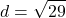General Formulas and Concepts:

Pre-Algebra

Order of Operations: BPEMDAS

1. Brackets
2. Parenthesis
3. Exponents
4. Multiplication
5. Division
7. Subtraction
• Left to Right

Algebra I

• Coordinates (x, y)

Algebra II

• Distance formula: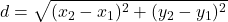Step-by-step explanation:

Step 1: Define

Point C(-5, -2)

Point D(0, -4)

Step 2: Find distance d

Simply plug in the 2 coordinates into the distance formula to find distance d

1. Substitute in points [Distance Formula]: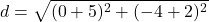2. [√Radical] (Parenthesis) Add: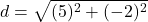3. [√Radical] Evaluate exponents: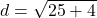4. [√Radical] Add: